### Human Eye And Colourful World Class 10th Physics & Chemistry AP Board Solution

##### Question 1.How do you correct the eye defect Myopia?Answer:Myopia or near-sightedness or short-sightedness is one such defect in which a person is unable to see far away things clearly. In this defect, a person can see objects placed at certain distance (far point) from the eye only but everything beyond that distance appears blurry. The maximum distance upto which a person can see things clearly that is image is formed on retina is called the far point. Some of the causes of myopia are:● Increase in the axial length of the eye, which eventually shifts the retina to a certain level.● Cornea/lens becomes too curved for the length of the eyeball.● If parents are myopic, there are high chances that child too can have this defect.Actually what happens in myopia is, the rays coming from the object, after refraction from the lens, converges to a point before the retina as retina is the screen where the image should be obtained. In other words, the focal length of the eye lens decreases. So the rays are focused before retina and the image is not obtained clearly leading to blurry image.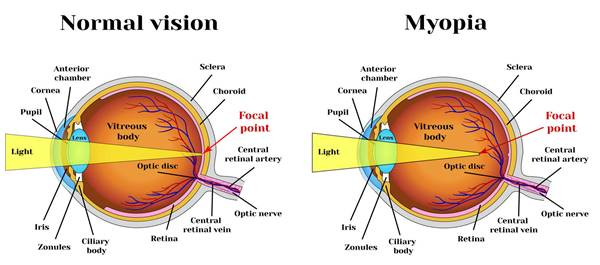Now the main objective is to focus the light on the retina. So a double-concave lens is used in front of the eye to fulfill this purpose. A double concave lens spread the light coming from the far away placed object that is makes the light rays parallel so that the rays converge at retina and thus forming a clear image. In other words, the concave lens brings the image of the object, placed beyond the far point, in between the far point & the least distance of distinct vision. Now this image acts an object for convex lens and image is formed on retina. The focal length, f of the lens should be equal to the negative of distance of far point,D.f = -D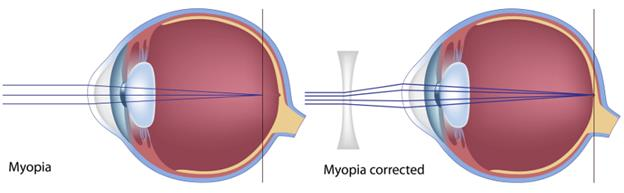Question 2.Explain the correction of the eye defect Hypermetropia.Answer:Hypermetropia or far sightedness is an eye defect in which a person is not able to see things placed closer to the eye. A normal person can see things clearly beyond 25 cm (least distance of distinct vision). If an object is placed between the least distance of distinct vision & the near point (the minimum distance upto which the objects can be seen clearly) then a person suffering from hypermetropia will not be able to see such things. Some of the causes of hypermetropia are:● Low converging power of eye lens because of weak action of ciliary muscles.● Abnormal shape of the cornea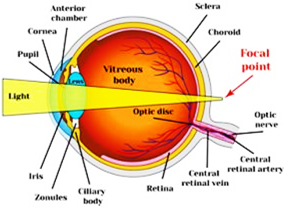In this defect, the light rays coming from the object placed between near point & the point of least distance of distinct vision, after refraction from the eye lens converges to a point beyond the retina. This implies that the image is formed beyond the eye thus creating a blurry image. In such cases the focal length of the eye lens increases. So to bring the image on retina a double convex lens is used. The double convex lens converge the light rays coming from the object in front of the eye lens. In other words, the convex lens creates an image of the object at the near point. Now this image acts as an object for the eye lens and thus forming image on the retina. The focal length of the convex lens should be:f = 25d/(d-25)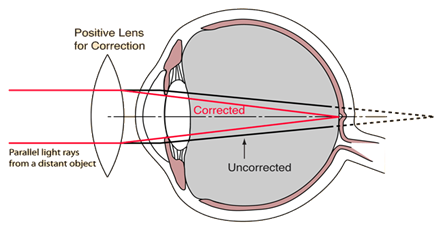Question 3.How do you find experimentally the refractive index of material of a prism.Answer:Objective : To find the refractive index of material of a prismMaterials required : prism, a white sheet, thumb pins, protractor, pencil, scaleFormula used : n =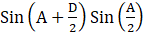where n = refractive index of material of prismA = angle of prismD = angle of deviationProcedure● Place the white sheet of paper on the table. Put the prism on the table such that the triangular base is on the white sheet.● Trace the triangular base on sheet with the help of pencil.● Now remove the prism. Name the triangle as P,Q & R.● With the help of protractor, find the angle between PQ & PR. It is the angle of prism (A).● On the side PQ, draw a perpendicular at point M.● Draw the incident ray at an angle say 30 degrees with respect to the normal and extend the line till point M. This angle is angle of incidence.● Place the prism in the same position as earlier on the trace of the triangular base.● Fix the pins at point A & B on the incident ray.● See the images of the pins A & B through the prism and fix pins at point C & D so that all the pins appear to be in same line.● Remove the prism and draw a line through points C & D. This line is the emergent line and the angle between emergent line & the normal is called angle of emergence.● Join the points M & N so that the path of light can be traced.● Now extend the incident ray & emergent ray till they meet at some point O. The angle between the two rays is called angle of deviation (D).● Repeat the above steps for various angles of incidence and note down the observations.● The refractive index can be calculated using the formula: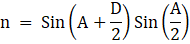● Plot the graph between angle of incidence & angle of deviation.Observation TableAngle of prism (A) = 60 degrees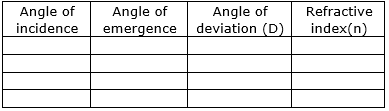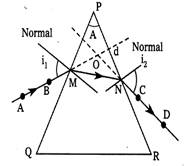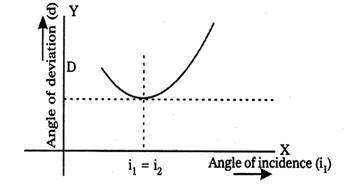Question 4.Explain the formation of rainbow.Answer:i. Sunlight is comprised of seven colours namely violet, indigo, blue, green, yellow, orange & red (VIBGYOR). Every colour has different wavelength such as red has the maximum wavelength & violet has the least wavelength.ii. As the sunlight travels in air (refractive index:1), all the colours travel with the same speed. But as soon as they travel in some medium, they get dispersed into seven colours as in this medium, the velocity of each colour changes. Rainbow is formed due to two phenomenons: reflection, dispersion & refraction.iii. Rainbow is seen mainly in rainy season as the spherical droplets of water that are suspended in air cause the dispersion of sunlight.iv. White light from the sun enters the top surface of the water droplet. Due to refraction, the sunlight disperses into seven colours & strikes the surface of droplet at point C.v. At point C, the angle formed by incident ray is greater than the critical angle. Therefore, total internal reflection occurs at point C and the dispersed spectrum of lights are reflected at the bottom surface of the droplet.vi. At point D, the spectrum again experiences refraction and as they are travelling from denser to rarer medium, the angle between red & violet increases as compared to the angle for the first refraction. There is one more condition, that is, bright rainbow is seen only when the angle between the incoming & the outgoing rays have the maximum angle of 42 degrees.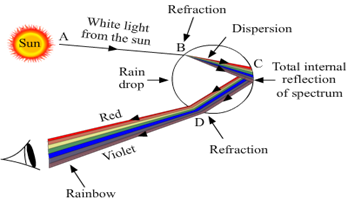Question 5.Explain briefly the reason for the blue of the sky.Answer:i. Sky appears blue due to a phenomenon called scattering of light. When a wavelength of certain frequency strikes the atom, the atom absorbs the energy and gets excited to another level.ii. Excited state is an unstable state, so in order to attain stability, the atoms comes back to the ground state releasing energy in form of light. This process occurs in scattering of light.iii. Scattering phenomenon occurs only when the size of the atom is comparable to the wavelength of light. Our atmosphere is comprised of oxygen and nitrogen molecules. So the wavelength of blue & violet is comparable to these molecules.iv. Therefore the molecules present in air scatter the blue & violet light. As human eye is sensitive to blue more than violet, therefore the sky appears blue Also the intensity of light depends on the angle of scattering. Intensity is maximum when angle of scattering is equal to 90 degrees. This is the reason why the sky appears more blue overhead than other parts.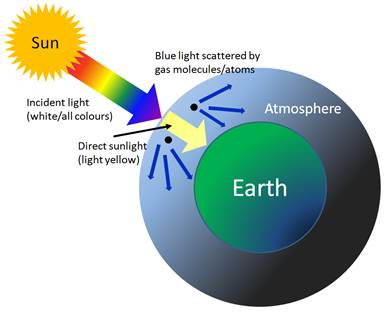Question 6.Explain two activities for the formation of artificial rainbow.Answer:Activity 1:● Select a wall on which the sun rays fall.● Stand in front of the wall facing the wall.● Hold a tube through which water is flowing.● Try to obstruct the flow of water in such a manner such that water comes out as fountain.● Hence rainbow is obtained when the rays of sunlight are dispersed by the water dropletsActivity 2:● Take a metal tray & fill it with water.● Place a mirror in the water such that it makes an angle to the water surface.● Now focus white light on the mirror through water.● Try to obtain colours on the white card board sheet kept above the water surface.Question 7.Derive an expression for the refractive index of the material of a prism.Answer: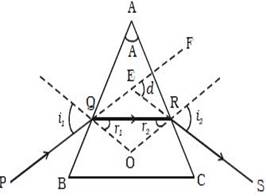Here ABC represents the triangular base of prismPQ = incident ray A = angle of prismOR = refracted rayRS = emergent rayd = angle of deviationFrom triangle EQR,d = i1 – r1 + i2 – r2d = (i1 + i2) – (r1 + r2 ) … (1)From triangle AQR,A +(90o – r1 ) + (90o – r2 ) = 180oA = r1 + r2 … (2)From (1) & (2)d = (i1 + i2) – Ad +A = (i1 + i2) … (3)Using Snell’s law, n1 sin I = n2 sin rUsing Snell’s Law At point M,n1 = refractive index of air = 1n2 = refractive index of material of prism = ni = i1 & r = r1sin i1 = n sin i2 …. (4)Using Snell’s law at N,n1 = nn2 = 1i = r2 & r = i2n sin r2 = sin i2 …(5)When i1 = i2, QR becomes parallel to BC and d becomes angle of minimum deviation (D).Then from equation (3)D +A = (i1 + i2) = 2i1i1 = D+A/2When i1 = i2, then r1 = r2From equation (2)2r1 = Ar1 = A/2Substituting the values in equation (4),Sin{(A+D)/2} = n sin (A/2)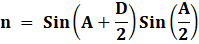Question 8.Light of wavelength enters a medium with refractive index n2 from a medium with refractive index n1. What is the wavelength of light in second medium?Answer:Refractive index in medium 1= n1 = c/v1Refractive index in medium 2 = n2 = c/v2Divide n1 by n2n1/n2 = v2/v1 …(1)Wavelength in medium 1 = λ1Wavelength in medium 2 = λ2Velocity of light in medium 1 = v1Velocity of light in medium 2 = v2According to the formula,v = λf where f = frequencyFor medium 1: v1 = λ1f …(2)For medium 2: v2 = λ2 f ….(3)divide equation (2) by (3)v1/ v2 = λ1/λ2 …(4)From equation (1) & (4)v1/ v2 = λ1/λ2 = n2/n1λ2 = (n1/n2) λ1Question 9.Choose the correct option by making hypothesis is based on given assertion and reason. Give an explanationAssertion (A): The refractive index of a prism depends only on the kind of glass of which it is made of and the colour of light.Reason (R): The refractive index of a prism depends on the refracting angle of the prism and the angle of the minimum deviation.A. Both A and R are true and R is the correct explanation of A.B. Both A and R are true and R is not the correct explanation of A.C. A is true but R is false.D. Both A and R are false.E. A is false but R is true.Answer:Refractive index refers to the ratio of velocity of light in vacuum to the velocity of light in medium. Yes refractive index depends on the material of glass used to construct prism as light passing through different materials have different velocities and thus refractive index also changes with material. Therefore, Assertion A is correct.According to the formula: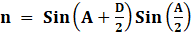We can infer that refractive index depends on the refracting angle (A) & the angle of minimum deviation (D). So, reason (R) is also correct.But, reason (R) is not the correct explanation of assertion(A) as material used to construct prism do not depend on the refracting angle (A) & the angle of minimum deviation (D).Question 10.Choose the correct option by making hypothesis is based on given assertion and reason. Give an explanationAssertion (A): Blue colour of sky appears due to scattering of light.Reason (R): Blue colour has shortest wavelength among all colours of white light.A. Both A and R are true and R is the correct explanation of A.B. Both A and R are true and R is not the correct explanation of A.C. A is true but R is false.D. Both A and R are false.E. A is false but R is true.Answer:Assertion (A) is correct because the sky appears blue due to scattering of light. Our atmosphere is comprised of oxygen and nitrogen molecules. So the wavelength of blue & violet is comparable to these molecules. Therefore the molecules present in air scatter the blue & violet light.Reason (R) is false as violet has the shortest wavelength among all the colours of white light. So, in the prism experiment it is deviated the most. As human eye is more sensitive to blue light than violet, therefore the shy appears blue to us.So, A is true & R is false.Question 11.Suggest an experiment to produce a rainbow in your classroom and explain the procedure.Answer:Objective: To produce a rainbow in classroomMaterials required : prism, wooden plank, white light source,tableProcedure:● Cover all the windows & close the doors so that the classroom becomes a dark room.● Take the prism & place it on the table in front of the white wall.● Take a wooden plank and make a small hole in it.● Keep the wooden plank in between the white light & the prism so that a narrow beam of light falls on the refracting surface of the prism.● Adjust the height of the prism so that the narrow beam of light falls on the refracting surface of prism.● Observe the changes in the emergent rays.● Now rotate the prism so that perfect spectrum is obtained on the wall.Conclusion: The spectrum of light is obtained on the wall of the classroom due to dispersion of light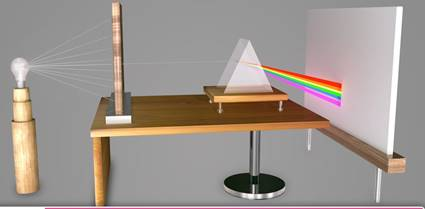Question 12.Prisms are used in binoculars. Collect information why prisms are used in binoculars.Answer: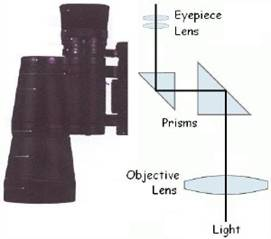● Binoculars are simply two telescopes side by side, one for each eye. When light rays from a distant object pass through a convex lens, they cross over. That's why distant things sometimes look upside down if we look at them through a magnifying glass.● Two objective lenses are situated at each end of the binoculars. The purpose of the objective lens is to collect light from the object that the user is looking at and bringing the collected light into focus in the eyepiece lens, which creates a visible and magnified image.● The image generated is backwards and inverted. Corrective elements known as prisms are used to fix this problem and they are situated between the objective lens and the eyepiece lens.● The prisms used in binoculars are basically blocks of glass that acts like mirrors. The prisms use internal reflections to bring the beam of light from the objective lens closer together and to correct the orientation of the image created by the objective lens.● Two types of prisms are used in binoculars: roof prisms and porro prisms.Question 13.Incident ray on one of the face (AB) of a prism and emergent ray from the face AC are given are given in figure Q.13. Complete the ray & diagram.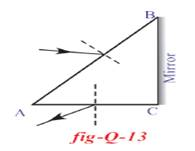Answer: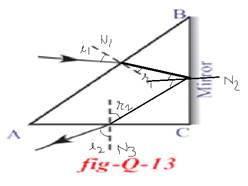AB & AC are refracting surfaces and BC is the reflecting surface. The incident ray strikes the surface AB. As the ray travels from air(rarer) to glass(denser), the ray bends towards the normal N1 & strikes surface BC. At BC, the ray gets reflected due to total internal reflection and strikes side AC. At AC, the ray travels from glass to air, therefore the ray moves away from the normal.Question 14.How do you appreciate the role of molecules in the atmosphere for the blue colour of the sky?Answer:The sky appears blue due to scattering phenomenon of light.Scattering phenomenon occurs only when the size of the atom is comparable to the wavelength of light. Our atmosphere is comprised of oxygen and nitrogen molecules. So the wavelength of blue & violet is comparable to these molecules. These molecules absorb light energy from the sunlight of certain frequency and molecules gets into excited state. When they come back to ground state, they emit light matching the wavelength of blue & violet. Therefore the molecules present in air scatter the blue & violet light. As human eye is sensitive to blue more than violet, therefore the sky appears blue Also the intensity of light depends on the angle of scattering. Intensity is maximum when angle of scattering is equal to 90 degrees. This is the reason why the sky appears more blue overhead than other parts.Question 15.Eye is the only organ to visualize the colourful world around us. This is possible due to accommodation of eye lens. Prepare a six line stanza expressing your wonderful feelings.Answer:My eyes see the world as bright!The oceans & the mountain’s heightThe colourful flowers & butterflyIt’s such a lovely sight…From greenery of forest to the beautiful rainbowOh eyes! you are only to see through!Question 16.How do you appreciate the working of Ciliary muscles in the eye?Answer:The ciliary muscles are a ring smooth muscle in the eyes middle layer that accommodates the focal length of the eye lens so that the eyes can see at various distances.● The ciliary muscles regulate the flow of aqueous humor.● It controls the accommodation of the eye lens.● When a person is trying to see far away objects, the ciliary muscles relaxes itself so that the lens elongates. This elongation of lens increased the focal length of the eye lens & thus we are able to see objects placed very far.● The ciliary muscles contract itself if a person has to see nearby objects. This makes the lens more curved which causes the focal length to shorten itself so the person can see nearby objects.● This is the reason why we are unable to see objects kept in room for a short time when we enter the room from outside. It takes a short time to accommodate the lens of eyes.Question 17.Why does the sky sometimes appear white?Answer:The sky sometimes appears white because our atmosphere is comprised of gas molecules such as oxygen, nitrogen as well as dust particles. The size of these molecules & atoms differ to a large extent. Also there is water vapour in the atmosphere whose size is much larger compared to the gas molecules. So what happens is, theses larger particles of dust & molecules of water vapour scatter the colours of other frequency except blue as the size of the atoms of dust & water molecules are not comparable with the wavelength of blue light. So the scattered light of all the other frequencies gets combined and reach our eyes and thus the sky appears white.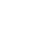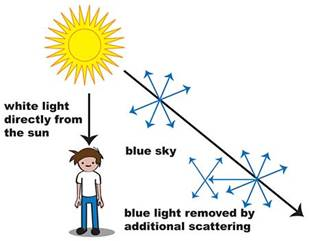Question 18.Glass is known to be a transparent material. But ground glass is opaque and white in colour. Why?Answer:Ground glass is a glass whose surface has been ground to produce a flat and rough surface. Glass is transparent as its surface is even in the molecular level resulting in transmission of light rays falling on it. In ground glass the surface is rough due to microscopic unevenness. So when light rays fall on it, then due to rough surface, they are reflected back in different direction instead of transmitting.Thus the ground glass is opaque and white in colour.Question 19.If a white sheet of paper is stained with oil. The paper turns transparent. Why?Answer:When white light falls on the paper, some rays gets transmitted, some get reflected, some absorbed and some scattered. Transparency of a material depends on the ratio of light transmitted to the light reflected. The more light is transmitted, the more is its transparency. Scattering of light depends on the size of the molecules as well as the difference in the refractive index of the two mediums. The paper contains molecules of larger size and is absorbent in nature. When dipped into oil, the oil molecules fit into the molecules of paper and fill in the voids in paper thus creating a path so that light can transmit through it with less scattering. Moreover oil lowers down the refractive index of paper thus increasing the transparency.Question 20.A light ray falls on one of the faces of a prism at an angle 40o so that it suffers angle of minimum deviation of 30°. Find the angle of prism and angle of refraction at the given surface.Answer:Given: Angle of incidence, i = 40oAngle of minimum deviation, D = 30oLet angle of prism be AUsing formula: A+D = 2iA = 2i-D =2(40o)-30o = 80o – 30o = 50oA = 50oUsing formula: r = A/2 (when angle of minimum deviation is considered)r = 50o/2 = 25oQuestion 21.The focal length of a lens suggested to a person with hypemetropia is 100cm. Find the distance of near point and power of the lens.Answer:A person suffering from hypermetropia uses a biconvex lens.Given: focal length of lens, f = 100 cmPower = 100/f = 100/100 = 1 dioptreLet the distance of near point be dusing formula: f = 25d/(d-25)100 = 25d/(d-25)100(d-25) = 25d100d – 2500 = 25d100d-25d = 250075d = 2500d = 2500/75 = 33.33 cmd = 33.33 cmQuestion 22.A person is viewing an extended object. If a converging lens is placed in front of his eye, will he feel that the size of object has increased? Why?Answer:If a convex lens is placed in front of eye then it will act as a dual convex lens.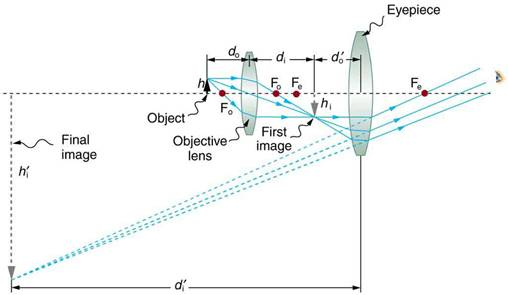The ray diagram would be like as shown in figure of microscope. Take the eyepiece as our eye. The light rays from the extended source strikes the objective lens and after refraction it will converge at beyond the focal length of eyepiece. This image will act as an object of the eyepiece which is able to accommodate its focal length according to the distance of the object and thus the final image formed is enlarged & inverted.Thus the person will be able to see an enlarged image of the object.

PDF FILE TO YOUR EMAIL IMMEDIATELY PURCHASE NOTES & PAPER SOLUTION. @ Rs. 50/- each (GST extra)

HINDI ENTIRE PAPER SOLUTION

MARATHI PAPER SOLUTION

SSC MATHS I PAPER SOLUTION

SSC MATHS II PAPER SOLUTION

SSC SCIENCE I PAPER SOLUTION

SSC SCIENCE II PAPER SOLUTION

SSC ENGLISH PAPER SOLUTION

SSC & HSC ENGLISH WRITING SKILL

HSC ACCOUNTS NOTES

HSC OCM NOTES

HSC ECONOMICS NOTES

HSC SECRETARIAL PRACTICE NOTES

# 2019 Board Paper Solution

HSC ENGLISH SET A 2019 21st February, 2019

HSC ENGLISH SET B 2019 21st February, 2019

HSC ENGLISH SET C 2019 21st February, 2019

HSC ENGLISH SET D 2019 21st February, 2019

SECRETARIAL PRACTICE (S.P) 2019 25th February, 2019

HSC XII PHYSICS 2019 25th February, 2019

CHEMISTRY XII HSC SOLUTION 27th, February, 2019

OCM PAPER SOLUTION 2019 27th, February, 2019

HSC MATHS PAPER SOLUTION COMMERCE, 2nd March, 2019

HSC MATHS PAPER SOLUTION SCIENCE 2nd, March, 2019

SSC ENGLISH STD 10 5TH MARCH, 2019.

HSC XII ACCOUNTS 2019 6th March, 2019

HSC XII BIOLOGY 2019 6TH March, 2019

HSC XII ECONOMICS 9Th March 2019

SSC Maths I March 2019 Solution 10th Standard11th, March, 2019

SSC MATHS II MARCH 2019 SOLUTION 10TH STD.13th March, 2019

SSC SCIENCE I MARCH 2019 SOLUTION 10TH STD. 15th March, 2019.

SSC SCIENCE II MARCH 2019 SOLUTION 10TH STD. 18th March, 2019.

SSC SOCIAL SCIENCE I MARCH 2019 SOLUTION20th March, 2019

SSC SOCIAL SCIENCE II MARCH 2019 SOLUTION, 22nd March, 2019

XII CBSE - BOARD - MARCH - 2019 ENGLISH - QP + SOLUTIONS, 2nd March, 2019

# HSCMaharashtraBoardPapers2020

(Std 12th English Medium)

HSC ECONOMICS MARCH 2020

HSC OCM MARCH 2020

HSC ACCOUNTS MARCH 2020

HSC S.P. MARCH 2020

HSC ENGLISH MARCH 2020

HSC HINDI MARCH 2020

HSC MARATHI MARCH 2020

HSC MATHS MARCH 2020

# SSCMaharashtraBoardPapers2020

(Std 10th English Medium)

English MARCH 2020

HindI MARCH 2020

Hindi (Composite) MARCH 2020

Marathi MARCH 2020

Mathematics (Paper 1) MARCH 2020

Mathematics (Paper 2) MARCH 2020

Sanskrit MARCH 2020

Sanskrit (Composite) MARCH 2020

Science (Paper 1) MARCH 2020

Science (Paper 2)

Geography Model Set 1 2020-2021

MUST REMEMBER THINGS on the day of Exam

Are you prepared? for English Grammar in Board Exam.

Paper Presentation In Board Exam

How to Score Good Marks in SSC Board Exams

Tips To Score More Than 90% Marks In 12th Board Exam

How to write English exams?

How to prepare for board exam when less time is left

How to memorise what you learn for board exam

No. 1 Simple Hack, you can try out, in preparing for Board Exam

How to Study for CBSE Class 10 Board Exams Subject Wise Tips?

JEE Main 2020 Registration Process – Exam Pattern & Important Dates

NEET UG 2020 Registration Process Exam Pattern & Important Dates

How can One Prepare for two Competitive Exams at the same time?

8 Proven Tips to Handle Anxiety before Exams!